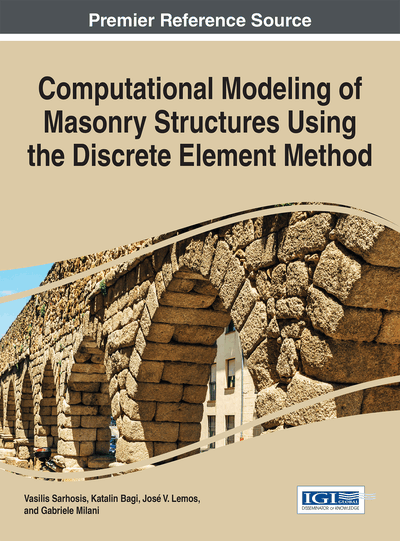# Introduction to the Combined Finite-Discrete Element Method

Máté Hazay (Budapest University of Technology and Economics, Hungary) and Ante Munjiza (Queen Mary University of London, UK)
DOI: 10.4018/978-1-5225-0231-9.ch006

## Abstract

This chapter presents a general overview of the combined Finite-Discrete Element Method (FEM/DEM) which is considered as a state-of-the-art technique for the mechanical analysis of masonry structures. In a FEM/DEM simulation each discrete element representing a stone block is discretized into finite elements in order to describe the deformability of the blocks. This chapter deals with the main steps of the FEM/DEM including contact detection, contact interaction, fracture and fragmentation algorithms, calculation of deformations and the time integration of the equation of motion. The FEM/DEM is advantageously used to simulate transition from continua to discontinua processes which may lead to the collapse of the structure. Some examples for practical applications found in the literature are mentioned.
Chapter Preview
Top

## Introduction

The two basic types of mechanical models are the classical models of continuum mechanics and the models of discrete elements. The finite element method (FEM) has been the most widely used technique to model continuum mechanical problems for decades. On the other hand, the discrete element method (DEM) is capable of describing discontinuum-based phenomena including the motion and interaction of individual particles. DEM was originally introduced by Cundall (Cundall, 1971) for modelling rock mechanical problems with the assumption that infinitely rigid blocks comprise the problem. Later, this pioneering model have been improved and modified by taking into account the deformability of the blocks. Such an alternative model is the discontinuous deformation analysis (DDA) introduced by Shi (Shi, 1988) where the blocks were able to deform uniformly. Intensive research work resulted in the appearance of several DEM codes, i.e. UDEC, YADE, etc. However in certain situations the continuum- and discontinuum-like phenomena arise at the same time, thus the development of a coupled numerical technique was required. Therefore, in the early 1990s the two above mentioned methods were combined and the resulting method was termed the combined finite-discrete element method (FEM/DEM). A typical combined finite-discrete element simulation comprises large number – thousands or even millions – of particles which are represented by a single discrete element. In FEM/DEM each discrete element is discretized into finite elements in order to describe the deformations of the blocks. Furthermore, the classical steps of discrete element method, including contact detection, contact interaction and time integration are applied to follow the motion and interaction of the individual particles. This method was implemented mainly to simulate so-called transition from continua to discontinua problems, including failure, fracture and fragmentation processes. Therefore, detailed structural collapse simulations can be performed with the help of the FEM/DEM. These simulations can play a vital role in the design of structures against hazardous loading conditions. In this case the load bearing capacity of the structure can be determined, and the progressive collapse modes can be identified as well. Some examples for modelling transition from continua to discontinua problems can be found at the end of this chapter and in (Munjiza, 2004, pp. 30-32).

Masonry is a heterogeneous structural material consisting of bricks joined by mortar layers. Numerical modelling of masonry structures - especially in the nonlinear range - represents an interesting challenge due to the extremely complex behaviour deriving from their semi-discrete, composite nature. Two different approaches exist for mechanical modelling of masonry structures, these are the micro modelling and macro modelling of masonry structures. In case of the macro modelling approach, the structure is handled as an orthotropic continuum and the constitutive relations are described with the help of experiments and homogenization techniques. The serious disadvantage of this continuum-based approach is its inability to describe large discontinuities which occur during the collapse process. In order to ease this restriction contact elements may be implemented into the finite element mesh. This approach enables to consider the material nonlinearity where the constitutive behaviour of the contact elements are prescribed according to the theory of plasticity or damage mechanics. Unfortunately, even with the application of contact elements, the finite element method is unable to handle the mechanical interaction of several blocks. Due to this fact, reliable collapse simulations including progressive failure modes cannot be carried out with the application of the FEM. However FEM/DEM includes contact detection and contact interaction algorithms, thus this technique is capable of modelling the mechanical interaction of several bodies. Therefore, numerical models based on the combined finite-discrete element method may be developed for analysing such problems.

## Complete Chapter List

Search this Book:
Reset